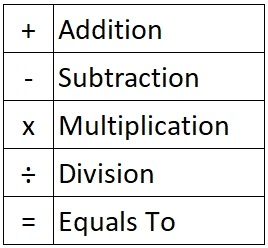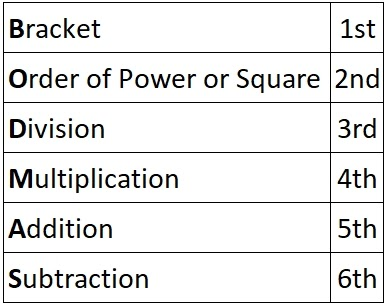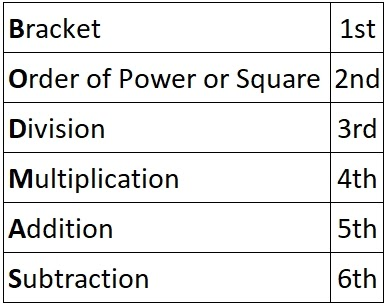Math Concepts
Introduction to Mathematical Operations
14.3k views

 1 Numbers and Symbols 2 Operators and Operands 3 BODMAS 4 Importance of BODMAS 5 BODMAS in detail 6 Worked out Examples 7 Practice Examples 8 Summary 9 Frequently Asked Questions (FAQs) 10 External References

19 January 2021

Before getting to know what Mathematical-operations are, one has to understand Operators and Operands. Every object in Maths represents either a value or a function.

## What are Numbers and Symbols?

Example:
1 represents a count of a single item.
For instance 1 chocolate,  1 box of chocolates, etc.,
And these mathematical objects are called numbers.

Numbers can now be defined as values, and each number will have its respective values.

Maths also has another set of objects, called the symbols.

Example:
As a part of learning, we all know that the symbol + represents ADD.
Similarly, -  is a symbol that represents the function SUB.
These symbols are functional by nature and define the operation they perform.

From the above,
NUMBERS are VALUES
While
SYMBOLS are FUNCTIONS

The detailed importance of mathematical operations is mentioned below. To view them click on the below Download button.

## What are Operators and Operands?

Knowing the difference between and purpose of Numbers/Values and Symbols/Functions, will help us understand the difference between Operators and Operands. Knowing the difference between and purpose of Operators and Operands, will help understand Mathematical-operations.

Let us first focus on Operators. Operators are Mathematical Symbols that emphasise on a particular action, like how we have verbs in language English. Example, Run, Sit, Walk represents actions in English. Similarly ADD, SUB, etc., represents actions in Maths. In Maths these actions are termed functions.

The basic Operators which stand as a foundation in Maths are,Next, let us move to understand what Operands are?
Operands can be defined as a NUMBER or VALUE upon which the FUNCTION will be applied.

Example
If 2 and 5 are two given numbers or values, and ADD is the function given. Then the given statement can be written as,
2 + 5 or 5+ 2.
The operator (+) ADD serves as a function or action upon the numbers 2 and 5.
Every number on which an action or function is performed is termed as OPERANDS.
In the above example 2 + 5,
+ stands as OPERATOR
2 and 5 stand as OPERANDs

Either by summing 2 with 5, or 5 with 2 results an outcome. This outcome is the answer for the operator acting upon the operands.
In this case, it is
2 + 5 is 7. The word ‘is’ can be replaced with a Mathematical Operator called EQUALS-TO represented as =.
2 + 5 = 7 or
5 + 2 = 7

## What is BODMAS?

BODMAS is the next challenging, yet wonderful functionality in Maths.

• It is a predefined rule based on which Mathematical-operations can be performed.
• It can be defined as below and priority of use moves from top to bottom.The above RULE should be memorised in order to work out a given Mathematical operation.

## What is the Importance of BODMAS in Math?

The below illustration emphasizes why BODMAS plays an important role and why it can not be ignored in Mathematical-operations.
4 + 3 x 2 = ?

First operation will be 4 + 3 = 7
So 4 + 3 x 2 becomes 7 x 2, which is equal to 14.

Now let us apply the BODMAS rule and work out the same example 4 + 3 x 2.
BODMAS represents,

• Brackets

In this example, there is no bracket used hence, Bracket is ignored

• Order of Power or Square

No Power or Square is used in the given example, thus this step is also ignored

• Division

No Division used, hence moving to next priority Multiplication

• Multiplication

The given example has a Multiplication operation

That is 3 x 2 in the given example

4 + 3 x 2

3 x 2 = 6

The given problem 4 + 3 x 2 becomes 4 + 6

The given example has a Addition operation

The given example 4 + 3 x 2 is simplified to 4 + 6, which carries a ADDITION function

So, 4 + 6 = 10

• Subtraction

There are no further operations to be performed, hence ignoring the Subtraction operation.

The answer for 4 + 3 x 2 as per BODMAS rule is 10,

Whereas 4 + 3 x 2 by a traditional left to right movement calculation is 14.

Both the methods yield 2 different results.
In order to avoid such confusions, a rule is in place which needs to be followed.
In this case, it is BODMAS

The above illustration should have given satisfactory evidence about how important BODMAS is and why it should not be ignored while performing Mathematical-operations.

## BODMAS in Detailed Form

Below are a series of examples that emphasises on each part of BODMAS rule.
Use the checklist## Worked out Examples

• 4 x (3 + 2) = ?

= 4 x 5
=20

• 32 + 5 = ?

=9 + 5
=14

• 6 ÷ 2 + 7 x 4 = ?

=3 + 7 x 4
=3 + 28
=31

• 4 + 6 - 7 + 3 = ?

=10 - 4
=6

• 4 + 64 x ( 30 ÷ 5 ) = ?

=4 + 64 x 6
=4 + 384
=388

## Practice Examples

1. 3 + 20 x 3
2. 25 - 5 ÷ ( 3 + 2 )
3. 10 + 6 x ( 1 + 10 )
4. ( 3 + 2 ) + 52

## Summary

We have understood that an operation is a function which takes zero or more input values (called operands) to a well-defined output value. The number of operands is the arity of the operation.

The most commonly studied operations are binary operations (i.e., operations of arity 2), such as addition and multiplication, and unary operations

The article has shared information on how to approach a given set of problems using the BODMAS rule in mathematical-operations.

Image Credits
Images provided by VJ Dream Works (www.vjdreamworks.in / [email protected])